# 复杂波场页岩气探区速度分析方法探讨A Discussion on Velocity Analysis Method of Shale Gas Exploration Area with Complex Wave Field

DOI: 10.12677/JOGT.2018.406117, PDF, HTML, XML, 下载: 459  浏览: 1,490

Abstract: The wave field of XC Shale Gas Exploration Area was complex, and the stacking velocity was difficult to obtain. The velocity analysis by superposition energy method could not meet the processing requirements. This paper combined the characteristics of seismic data in the XC Shale Gas Exploration Area, focused on the impact of factors such as low signal-to-noise ratio and near-surface anomalies on the quality of the velocity spectrum, the targeted processing techniques and methods were proposed, a set of methods for precise extraction of seismic stack velocity in XC Shale Gas Exploration Area were summarized. Firstly, the original data were purified and the noise was suppressed by multi-domain and multi-method combination, and the signal-to-noise ratio was improved. Secondly, various residual static correction methods were used for maximally reducing the problem of high frequency static correction and improving the quality of seismic data. Finally, the relevant characteristics of instantaneous phase and the statistical phase correlation method were used to calculate the velocity spectrum, improve the precision of calculating speed. Better imaging results are obtained; especially the quality of structural imaging in the middle and deep layers is obviously improved.

1. 引言

2. 速度分析基本原理

2.1. 叠加能量法

$A=\frac{1}{N}{\sum }_{j=1}^{M}\left({\sum }_{i-1}^{N}{f}_{i,j+{r}_{i}}\right)$ (1)

2.2. 统计相位相关法

1) 设输入的道集为uij，表示第i道、第j个采样点。设初始时间为t0，选定其对应的速度v进行动校正，此时可以得到校正后的道集，再应用Hilbert变换法求取uij的瞬时振幅Aij和瞬时相位μij

2) 将整个道集分为若干部分，即样点分布分为c组，样点的相位表示为 ${\mu }_{is}\left(i=1,2,\cdots ,{n}_{s};s=1,2,\cdots ,c\right)$ ，且 ${\sum }_{s=1}^{c}{n}_{s}=M$ ，求取统计量R和W：

$R=\sqrt{{\left({\sum }_{s=1}^{c}{U}_{s}\right)}^{2}+{\left({\sum }_{s=1}^{c}{W}_{s}\right)}^{2}}$ (2)

$W=2\left({\sum }_{s=1}^{c}\frac{{R}_{s}^{2}}{{n}_{s}}-\frac{{R}^{2}}{M}\right)$ (3)

R假设检验与W假设检验是判断t0及所选定的v正确与否的标准。当R检验假设概率分布是单峰分布时，代表t0及所选定的v正确；当W检验假设概率分布在不同偏移距范围内，且都是相同的单峰分布时，代表t0及所选定的v正确。

3) 计算常规叠加能量的瞬时信息SB(t, v)：

${S}_{\text{B}}\left(t,v\right)=\frac{{\sum }_{i=1}^{M}B\left[{\sum }_{j=1}^{N}{u}_{ij}\left(t,v\right)\right]}{{\sum }_{i=1}^{M}L\left\{{\sum }_{j=1}^{N}B\left[{u}_{ij}\left(t,v\right)\right]\right\}}$ (4)

4) 计算能量函数SRWB

${S}_{\text{RWB}}=\left(\frac{R}{W}\right){S}_{\text{B}}$ (5)

3. 应用实例效果分析

3.1. 速度分析的预处理

3.1.1. 提高信噪比处理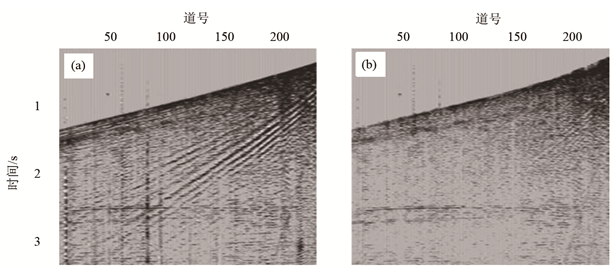Figure 1. The single shot result before (a) and after (b) denoising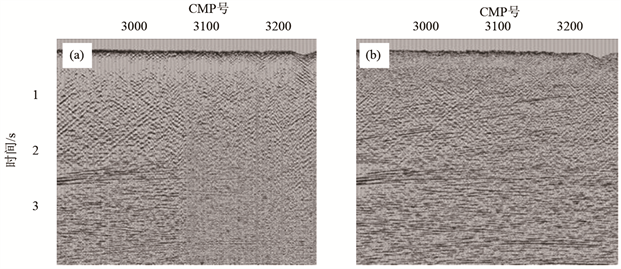Figure 2. The profile correlation before (a) and after (b) denoising

3.1.2. 剩余静校正处理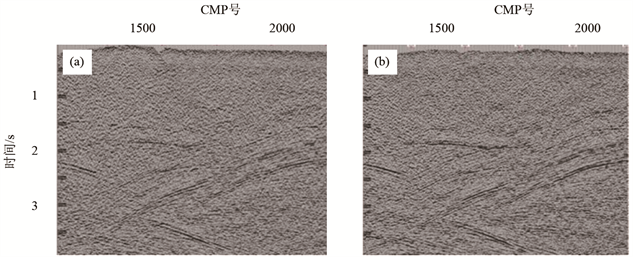Figure 3. The profile correlation before (a) and after (b) residual static calibration

3.2. 统计相位相关法速度谱的求取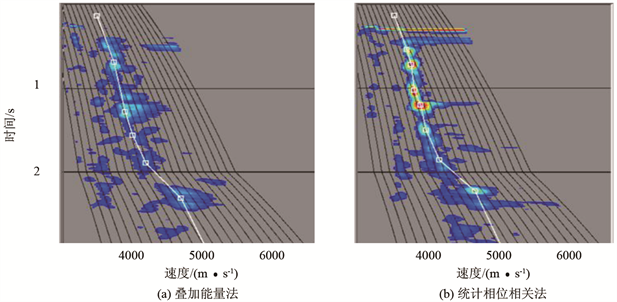Figure 4. The velocity spectrums of different velocity analysis methods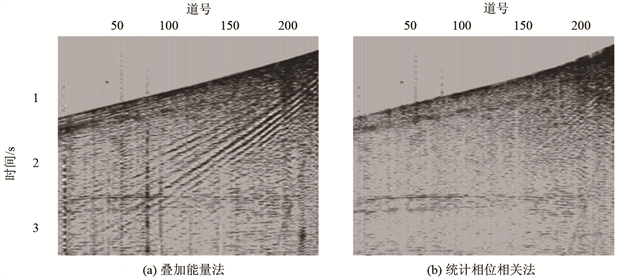Figure 5. The stack profiles of different velocity analysis methods

4. 结语

  李振春, 张军华. 地震数据处理方法[M]. 东营: 中国石油大学出版社, 2004: 1-308.  李庆忠. 走向精确勘探的道路——高分辨率地震勘探系统工程剖析[M]. 北京: 石油工业出版社, 1993: 70-80.  潘继平, 乔德武, 李世臻, 等. 下扬子地区古生界页岩气地质条件与勘探前景[J]. 地质通报, 2011, 30(2): 1671-2552.  Turhan, T.M. and Koehler, F. (1969) Velocity Spectra-Digital Computer Derivation and Applications of Velocity Functions. Geophysics, 34, 859-881. https://doi.org/10.1190/1.1440058  De Vries, D. and Berkhout, A.J. (1984) Velocity Analysis Based on Minimum Entropy. Geophysics, 49, 212-2142. https://doi.org/10.1190/1.1441629  Biondi, B.L. and Kostov, C. (1989) High-Resolution Velocity Spectra Using Eigenstruture Methods. Geophysics, 54, 832-842. https://doi.org/10.1190/1.1442712  Simon, K. (1991) Asymptotically Linear Velocity Analysis with High Resolution in Time Domain. Geophysics, 56, 1840-1848. https://doi.org/10.1190/1.1442997  吴树初, 罗国安. 相关速度谱和复数道相关速度谱[J]. 石油地球物理勘探, 1990, 25(3): 333-341.  林小竹. 协方差速度谱[J]. 石油地球物理勘探, 1993, 28(4): 403-410.  Byun, B.S., Corrigan, D. and Gaiser, J.E. (1989) Anisotropic Velocity Analysis for Lithology Discrimination. Geophysics, 54, 1564-1574. https://doi.org/10.1190/1.1442624  Alkhalifah, T. (1997) Velocity Analysis Using Nonhyperbolic Moveout in Transversely Isotropic Media. Geophysics, 62, 1839-1854. https://doi.org/10.1190/1.1444285  Huub, D. and Alexander, C. (2006) Nonhyperbolic Moveout Analysis in VTI Media. Geophysics, 71, D59-D71. https://doi.org/10.1190/1.2194901  Brahim, A., Bjorn, U. and Didier, R. (2009) Automatic Nonhyperbolic Velocity Analysis. Geophysics, 74, U1-U12.  Sergey, F. and Alexey, S. (2010) Generalized Nonhyperbolic Moveout Approximation. Geophysics, 75, U9-U18. https://doi.org/10.1190/1.3334323  徐翠娥, 郝晓红, 王影. 高密度双谱分析法在各向异性介质速度分析中的初步应用[J]. 海洋石油, 2008, 28(1): 1-5.  Morozov, L.B. and Smithson, S.B. (1996) High-Resolution Velocity Determination: Statistical Phase Correlation and Image Processing. Geophysics, 61, 1115-1127. https://doi.org/10.1190/1.1444032  王德利, 何樵登, 韩立国. 相位相关统计高分辨率速度分析[J]. 石油地球物理勘探, 2001, 36(2): 198-204.  张军华, 王静, 郑旭刚, 等. 关于几种速度分析方法的讨论及效果分析[J]. 石油物探, 2009, 48(4): 347-353.  谭胜章, 杜惠平, 宋国良, 等. 高精度三维地震资料采集技术[J]. 石油物探, 2007, 47(1): 74-80.  王西文, 赵邦六. 地震资料采集方式对地震处理的影响研究[J]. 地球物理学进展, 2010, 25(3): 840-852.  胡英, 张研, 陈立康, 等. 速度建模的影响因素与技术对策[J]. 石油物探, 2006, 45(5): 503-507.  郭树祥. 高分辨率地震资料处理中的优化速度分析方法[J]. 石油物探, 2004, 43(1): 80-82.  De Bazelaire, E. (1988) Nomudmoveou Revisited: Inhomogeneous Media and Curved Interfaces. Geophysics, 53, 143-157. https://doi.org/10.1190/1.1442449